## OpenGL学习脚印：深度測试(depth testing)

• 为什么须要深度缓冲区？
• OpenGL中怎么使用深度缓冲区 ？
• 可视化深度值
• 深度值的精度问题-ZFighting

# 问题背景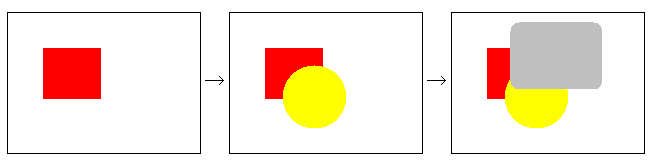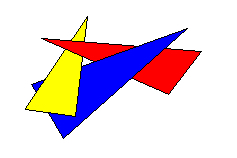# OpenGL中使用深度測试

   glEnable(GL_DEPTH_TEST);

   glClearColor(0.18f, 0.04f, 0.14f, 1.0f);
glClear(GL_COLOR_BUFFER_BIT | GL_DEPTH_BUFFER_BIT);

GL_ALWAYS 总是通过測试
GL_NEVER 总是不通过測试
GL_LESS 在当前深度值 < 存储的深度值时通过
GL_EQUAL 在当前深度值 = 存储的深度值时通过
GL_LEQUAL 在当前深度值 <= 存储的深度值时通过
GL_GREATER 在当前深度值 > 存储的深度值时通过
GL_NOTEQUAL 在当前深度值 不等于 存储的深度值时通过
GL_GEQUAL 在当前深度值 >= 存储的深度值时通过

  glDepthFunc(GL_ALWAYS);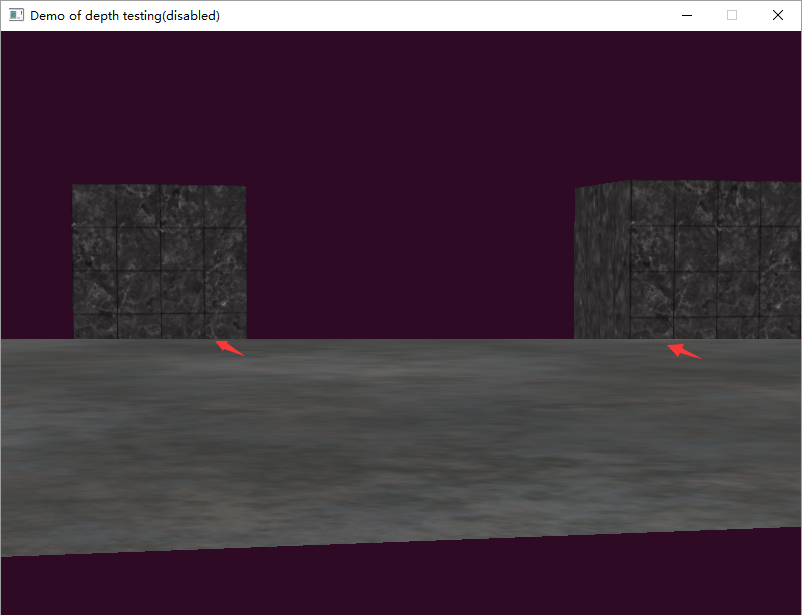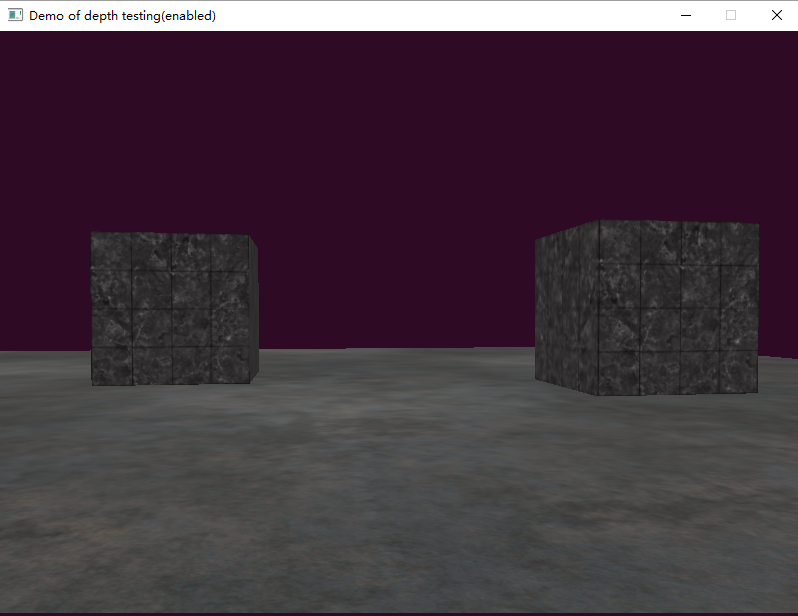# 可视化深度值

zwin 我们怎样获取呢？ 能够通过着色器的输入变量gl_FragCoord.z来获取。这个gl_FragCoord的z坐标表示的就是深度值。

  // 原样输出
float asDepth()
{
return gl_FragCoord.z;
}
void main()
{
float depth = asDepth();
color = vec4(vec3(depth), 1.0f);
}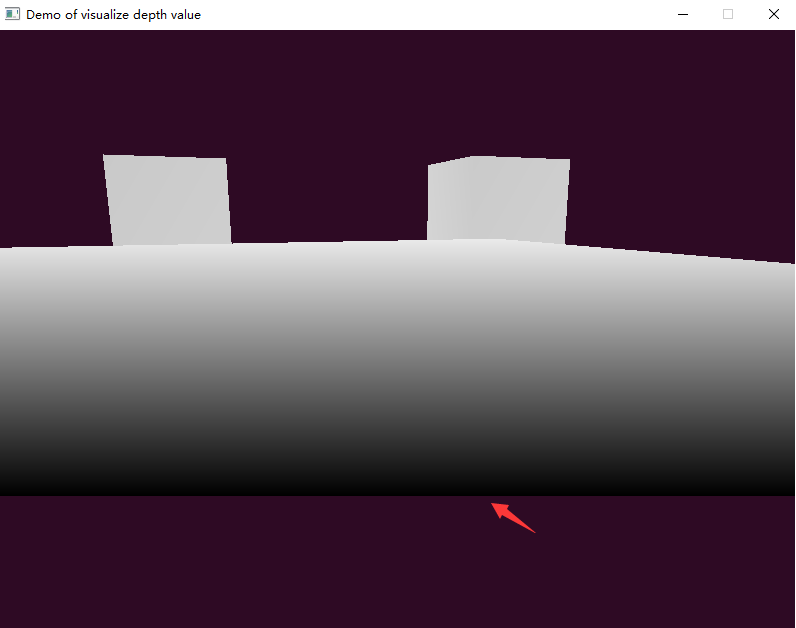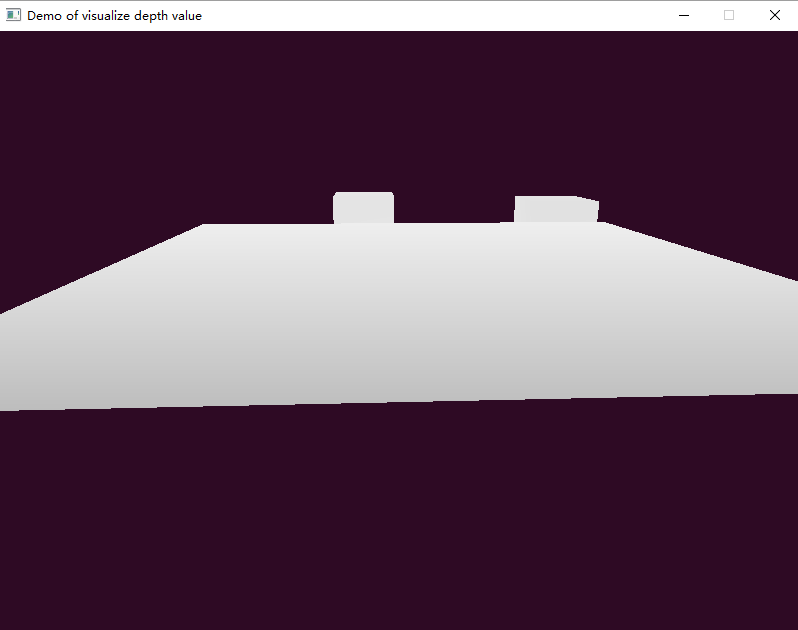zndc=f+nfnzeye2fnfnzeye=f+nfn+2fn(fn)zeye(1)

zwin=fsns2zndc+fs+ns2(2)

zwin=12zndc+12(3)
zndc=2zwin1(4)

zeye=2fnzndc(fn)(f+n)(5)

zeye=2fn(f+n)zndc(fn)(6)


// 线性输出结果
float near = 1.0f;
float far  = 100.0f;
float LinearizeDepth()
{
// 计算ndc坐标 这里默认glDepthRange(0,1)
float Zndc = gl_FragCoord.z * 2.0 - 1.0;
// 这里分母进行了反转
float Zeye = (2.0 * near * far) / (far + near - Zndc * (far - near));
return (Zeye - near)/ ( far - near);
}
void main()
{
float depth = LinearizeDepth();
color = vec4(vec3(depth), 1.0f);
}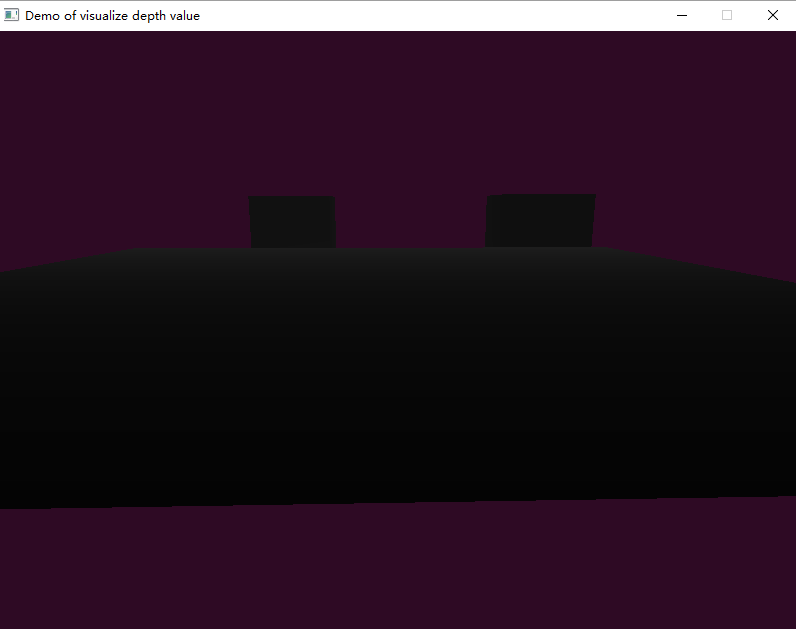zwin=1n1zeye1n1f(7)

   // 非线性输出
float nonLinearDepth()
{
float Zndc = gl_FragCoord.z * 2.0 - 1.0;
float Zeye = (2.0 * near * far) / (far + near - Zndc * (far - near));
return (1.0 / near - 1.0 / Zeye) / (1.0 / near - 1.0 / far);
}
void main()
{
float depth = nonLinearDepth();
color = vec4(vec3(depth), 1.0f);
}

# 深度的准确度问题-ZFighting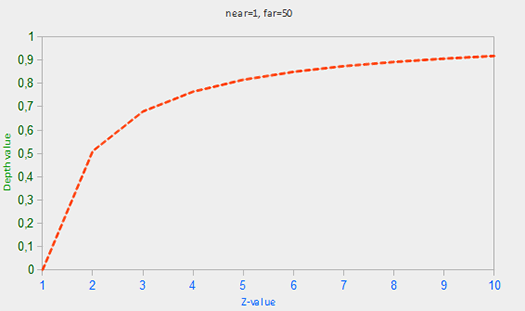zwin=S(fn(fn)zeye+12f+nfn+12)(8)

zwin=1=>zeye=fn/((1/s)(fn)f)
zwin=S1=>zeye=fn/(((s1)/s)(fn)f)

zwin=1=>zeye=0.01000015
zwin=S1=>zeye=395.90054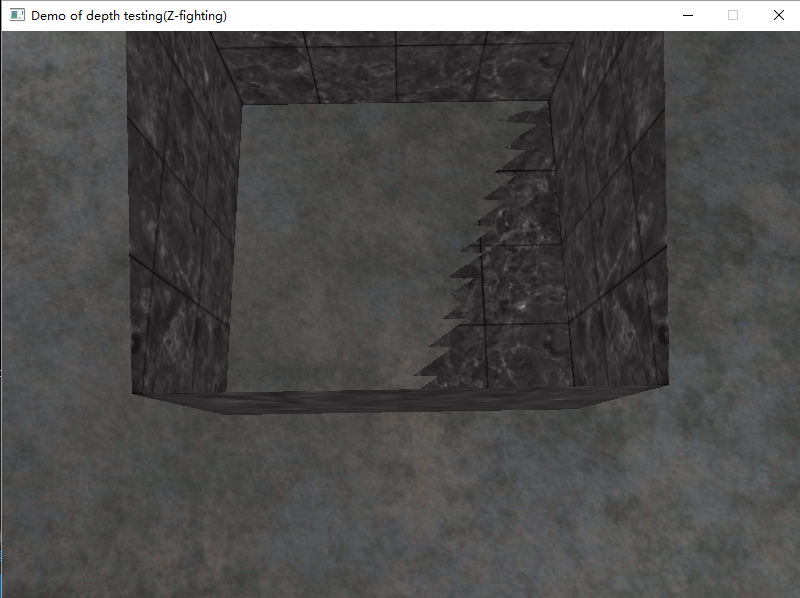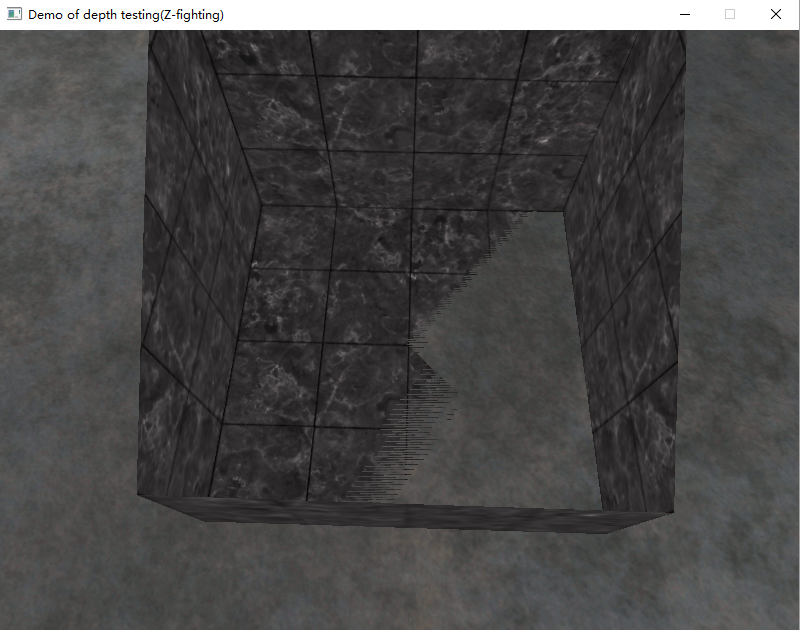（假设你要亲自观察这个现象，仅仅须要在本节代码中。将相机位置放在立方体内部。略微调整鼠标观察角度就能够了）。

# 预防ZFighting的方法

1.不要将两个物体靠的太近，避免渲染时三角形叠在一起。这样的方式要求对场景中物体插入一个少量的偏移，那么就可能避免ZFighting现象。

2.尽可能将近裁剪面设置得离观察者远一些。上面我们看到，在近裁剪平面附近，深度的准确度是非常高的，因此尽可能让近裁剪面远一些的话。会使整个裁剪范围内的准确度变高一些。可是这样的方式会使离观察者较近的物体被裁减掉，因此须要调试好裁剪面參数。
3.使用更高位数的深度缓冲区，通常使用的深度缓冲区是24位的，如今有一些硬件使用使用32位的缓冲区，使准确度得到提高。